FreeBuf+小程序2020-01-07 09:00:19

## Keras介绍

Keras是由 Python 编写的神经网络库，专注于深度学习，运行在 TensorFlow 或 Theano 之上。TensorFlowTheano是当前比较流行的两大深度学习库，但是对初学者来说相对有些复杂。Keras 使用简单，结构清晰，底层计算平台可基于 TensorFlow 或 Theano 之上，功能强大。Keras 可运行于 Python 2.7 或 3.5 环境，完美结合于 GPU 和 CPU，基于 MIT license 发布。Keras 由 Google 工程师François Chollet开发和维护。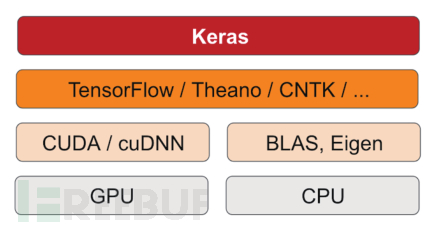## 安全能力&感知攻击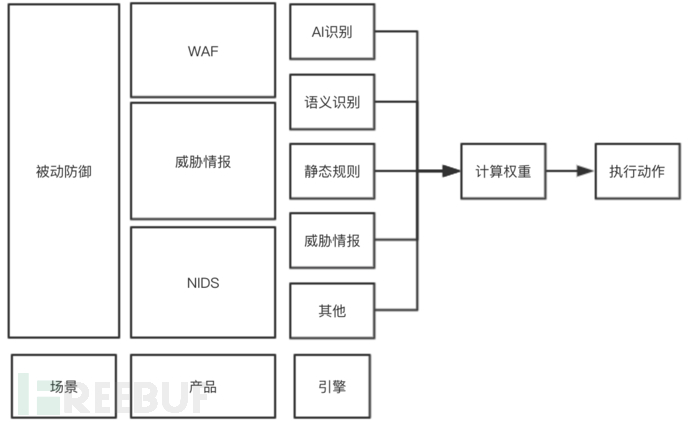## 实现思路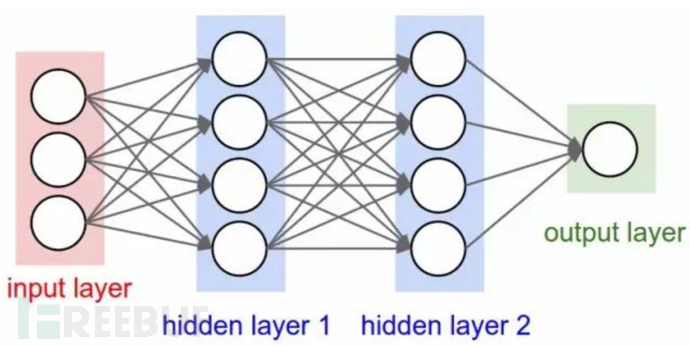``恶意流量：/examples/jsp/jsp2/el/search=<SCript>alert('xss')</scRIpt>恶意流量：/iajtej82.dll?<img src='javascript:alert(cross_site_scripting.nasl);'>恶意流量：/examples/jsp/jsp2/el/search=<prompt>alert(/1/)</prompt>恶意流量：/cgi-bin/index.php?gadget=glossary&action=viewterm&term=<script>alert('jaws_xss.nasl');</script>恶意流量：/fmnveedu.htm?a=<marquee loop=1 width=0 onfinish=confirm(1)>0</marquee>``

``<script>co\u006efir\u006d`1`</script>    <ScRiPt>co\u006efir\u006d`1`</ScRiPt>    <img src=x onerror=co\u006efir\u006d`1`>    <svg/onload=co\u006efir\u006d`1`>    <iframe/src=javascript:co\u006efir\u006d%28 1%29>    <h1 onclick=co\u006efir\u006d(1)>Clickme</h1>    <a href=javascript:prompt%28 1%29>Clickme</a>    <a href="javascript:co\u006efir\u006d%28 1%29">Clickme</a>    <textarea autofocus onfocus=co\u006efir\u006d(1)>    <details/ontoggle=co\u006efir\u006d`1`>clickmeonchrome    <p/id=1%0Aonmousemove%0A=%0Aconfirm`1`>hoveme    <img/src=x%0Aonerror=prompt`1`>    <iframe srcdoc="&lt;img src&equals;x:x onerror&equals;alert&lpar;1&rpar;&gt;">    "><h1/ondrag=co\u006efir\u006d`1`)>DragMe</h1>``

``def handle(self, payload):            payload = urllib.parse.unquote(payload.lower().strip())    # 数字泛化为"0"            payload = re.sub("\d+", '0', payload)    # 分词            r = '''                    (?x)[\w\.]+?\(                    |\)                    |"\w+?"                    |'\w+?'                    |http://\w                    |</\w+>                    |<\w+>                    |<\w+                    |\w+=                    |>                    |[\w\.]+                '''    """    原始链接            /0_0/e/data/ecmseditor/infoeditor/epage/tranflash.php?a=0"><script>alert(/0/)</script>< 1    分词链接            ['0_0/e/data'/ecmseditor/infoeditor/epage/tranflash.php？', 'a=', '0', '>', '<script>', 'alert(', '0', ')', '</script>']            """            nltks = nltk.regexp_tokenize(payload, r)            temp = []            for item in nltks:                if len(item) <= 3 or len(item) >= 10:                    continue                else:                    for char in item:                        temp.append(ord(char))            return temp``

``b1498592370545=1&v=13111002&COLLCC=3442798258&    t=check&rec=stratus&etyp=connect&zone=zibo5_cnc&url=119.188.143.32&errCnt=327&uid=d0a47beafc75e1549c7fdc23530fd959&uif=CNC|BeiJing-114.251.186.13&tvid=7706069409&defi=2&dlod=1&darea=1&ppapi=false&trkip=119.188.143.32&trkon=0&ver=3.1.0.15&dur=36431783    cn_600022,cn_600516,cn_000002,cn_600519,cn_000651,cn_600887,cn_002415,cn_601288,cn_000333,    _=1498179095094&list=sh600030    q=marketStat,stdunixtime&_=1498584939540    _=1498584888937/&list=FU1804,FU0,FU1707,FU1708,FU1709,FU1710,FU1711,FU1712    callback=_ntes_quote_callback54388229    _=1498552987540&list=hf_OIL``

`` def run(self):            gx = []            bx = []            gy = []            by = []            for data in c.load(goodXss):                data = c.handle(data)                if len(data) == 0:                    continue                else:                    gx.append(data)                    gy.append(0)            for data in c.load(badXss):                data = c.handle(data)                if len(data) == 0:                    continue                else:                    bx.append(data)                    by.append(1)            x_train, x_test, y_train, y_test = train_test_split(gx + bx, gy + by, test_size=0.3, random_state=100)            y_train = keras.utils.to_categorical(y_train, num_classes=2)            y_test = keras.utils.to_categorical(y_test, num_classes=2)            x_train = pad_sequences(x_train, maxlen=50, value=0.)            x_test = pad_sequences(x_test, maxlen=50, value=0.)            c.train(x_train, x_test, y_train, y_test)``

## 先了解什么是卷积神经网络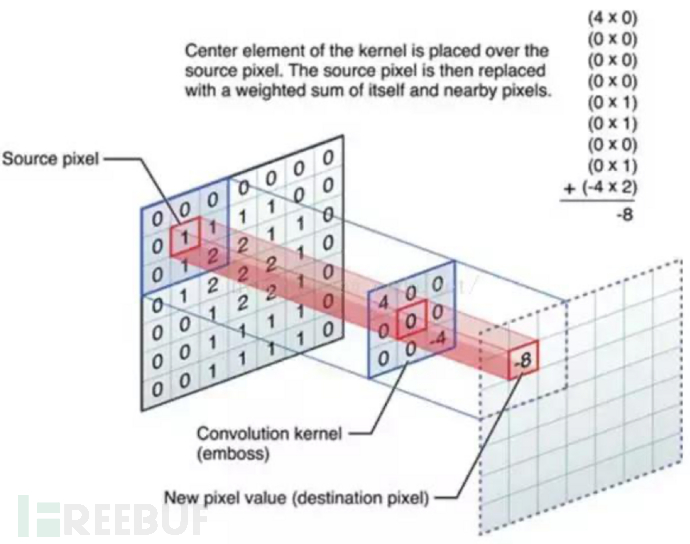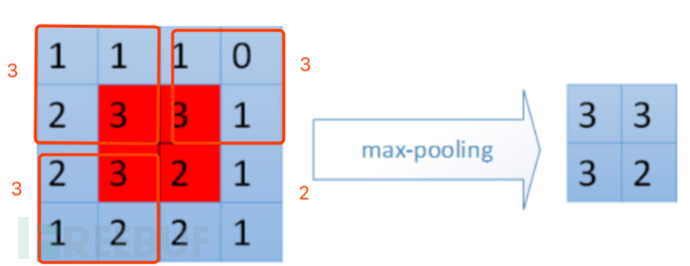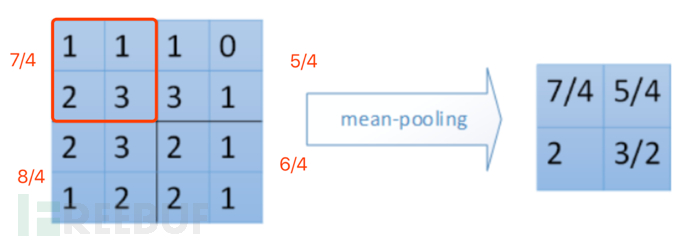`` def train(self, x_train, x_test, y_train, y_test):            model = self.NnModel()            OPTIMIZER = Adam(lr=0.001, beta_1=0.9, beta_2=0.999, epsilon=1e-08)            model.compile(loss="categorical_crossentropy", optimizer=OPTIMIZER,                          metrics=["accuracy", keras_metrics.precision(), keras_metrics.recall()])            model.summary()            model_name = "xss-cnn-32x2-{}".format(int(time.time()))            tensorboard = TensorBoard(log_dir='./'.format(model_name))            model.fit(x_train, y_train, batch_size=128, epochs=3, callbacks=[tensorboard])            train_score = model.evaluate(x_train, y_train, verbose=0)            print('Train loss:', train_score)            print('Train accuracy:', 100 * train_score)            test_score = model.evaluate(x_test, y_test, verbose=0)            print('Test loss:', test_score)            print('Test accuracy:', 100 * test_score)            model.save(model_name)``

``   def CnnModel(self, input_shape):            model = Sequential()            model.add(Embedding(input_dim=65025,                                output_dim=50,                                input_length=50))            model.add(BatchNormalization())            model.add(Conv1D(filters=32, kernel_size=3, strides=1, activation='relu'))            model.add(BatchNormalization())            model.add(Conv1D(filters=64, kernel_size=3, strides=1, activation='relu'))            model.add(BatchNormalization())            model.add(Conv1D(filters=128, kernel_size=3, strides=1, activation='relu'))            model.add(MaxPooling1D(pool_size=2))            model.add(Flatten())            model.add(Dense(2, activation='softmax'))            return model``

## 预测

``    def perdict(self, modelName, playload):            model = keras.models.load_model(modelName)            pd = self.handle(playload)            playloads = pad_sequences([pd], maxlen=50, value=0.)            result = model.predict_classes(playloads, batch_size=1)            print("XSS==>" if result ==  else "None==>", playload)       if __name__ == '__main__':        badXss = "./badxss.txt"        goodXss = "./goodxss.txt"        testXss = "./test-xss.txt"        num_classes = 2        c = CNNXSS()        c.run()        list = [x.strip().lower() for x in open(testXss).readlines()]        for k, v in enumerate(list):            c.perdict('xss-cnn-32x2-1577157928.h5', v.strip())``

## 后话

*本文原创作者：邹先生007，本文属于FreeBuf原创奖励计划，未经许可禁止转载

# xss # 神经网络 # keras

+ 收入我的专栏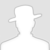• 0 文章数
• 0 评论数
• 0 关注者